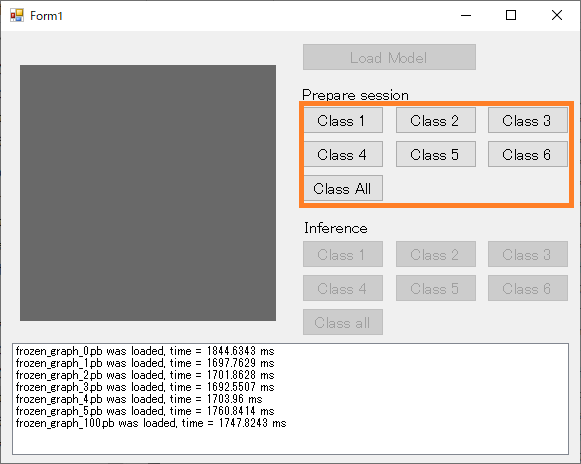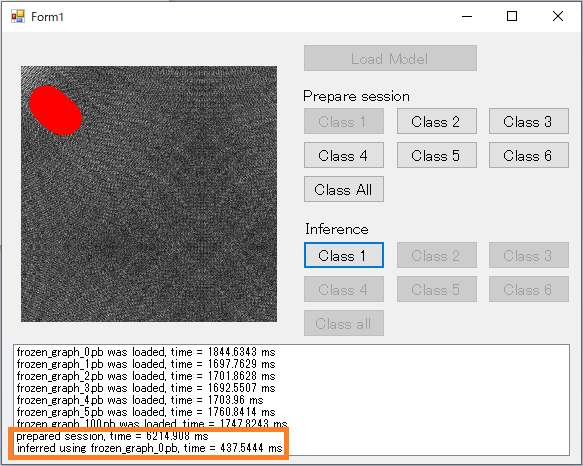# Accelerate inference by running tensorflow's session in advance

Hi, this is chang. Today, I tried to accelerate inference.

I published defection viewer not long ago*2*3. Its inference took about 6 sec. I investigated why it took so long and discovered that the time was costed in running a session of Tensorflow.

0. What is Session?

Tensorflow has unique mechanisms called graph and session. According to this*4:

• graph: it defines the calculation. It doesn’t compute anything and doesn’t hold any values.
• session: it allows to execute graphs or part of graphs. It allocates resources (on one or more machines) for that and holds the actual values of intermediate results and variables

To be honest, I had written source coeds without underestanding. Fact, it is not required if you use keras. I guess they are superficially removed in Tensorflow 2 because it standardize the interface of keras.

Tensorflow is a library of Python so we don't compile its source coeds. But in actual computation, I guess it loads the graph (=source codes) into memory as session.

1. First run costs???

For operating inference, you have to run session as below:

inference.cpp

``` /* run session */
TF_SessionRun(sess,
nullptr, // Run options.
&input_op, &input_tensor, 1, // Input tensors, input tensor values, number of inputs.
&out_op, &output_tensor, 1, // Output tensors, output tensor values, number of outputs.
nullptr, 0, // Target operations, number of targets.
status // Output status.
);```

Debugger showed that the "TF_SessionRun" took about 6 sec., so I misunderstood that every inference takes 6 sec. I felt something and tried to repeat run using for loop. The result showed only first step took 6 sec. and second or later ended just in 500 msec. I think the first run loads the program into memory and second or later reuse the region.

I wrote the function to run a session using empty date in advance:

inference.cpp

```int prepareSession(int model_num, int channel)
{
//  /* prepare input tensor */
TF_Output input_op = { TF_GraphOperationByName(graph[model_num], "input_1"), 0 };
if (input_op.oper == nullptr) {
return -2;
}

TF_Tensor* output_tensor = nullptr;

/* prepare session */
status = TF_NewStatus();
options = TF_NewSessionOptions();
sess[model_num] = TF_NewSession(graph[model_num], options, status);
TF_DeleteSessionOptions(options);

if (TF_GetCode(status) != TF_OK) {
TF_DeleteStatus(status);
return -3;
}

const std::vector<std::int64_t> input_dims = { 1, IMG_SIZE, IMG_SIZE, channel };
std::vector<float> input_vals(IMG_SIZE*IMG_SIZE*channel);
TF_Tensor* input_tensor = tf_utils::CreateTensor(TF_FLOAT,
input_dims.data(), input_dims.size(),
input_vals.data(), input_vals.size() * sizeof(float));

/* prepare output tensor */
TF_Output out_op = { TF_GraphOperationByName(graph[model_num], "conv2d_23/Sigmoid"), 0 };
if (out_op.oper == nullptr) {
return -4;
}

/* run session */
TF_SessionRun(sess[model_num],
nullptr, // Run options.
&input_op, &input_tensor, 1, // Input tensors, input tensor values, number of inputs.
&out_op, &output_tensor, 1, // Output tensors, output tensor values, number of outputs.
nullptr, 0, // Target operations, number of targets.
status // Output status.
);
return 0;
}```

You can start the function by pushing "Prepare session" button of viewer.2. Result

I pushed "Prepare session" button, "Inference" button in turn. Running session in advance reduced inference time to 500 msec.If you run session in background, for example in starting application, you can show as accelerating inference.

3. Limitation

There's a limitation. If you want to use multiple models like this trial, you have to run a session for every model. That means every model cost 6 sec for preparation. In addition, memory consumption is huge.

In this trial, the network architectures are completely same among models. Definition of input and output node is common like the followings:

inference.cpp

``` /* prepare input tensor */
TF_Output input_op = { TF_GraphOperationByName(graph[model_num], "input_1"), 0 };
if (input_op.oper == nullptr) {
return -2;
}```
``` TF_Output out_op = { TF_GraphOperationByName(graph[model_num], "conv2d_23/Sigmoid"), 0 };
if (out_op.oper == nullptr) {
return -4;
}```

In logical, the program can reuse the session if the library can change the values of weight ans bias loaded in memory. It reduces the time for preparation and memory consumption. Unfortunately, Tensorflow isn't likely to have the function. I hope improvement in future.

4. Afterword

It is possible that Tenserflow 2.0 no longer require the technique introduced in this article. I will investigate in near future.

I updated the source codes*5